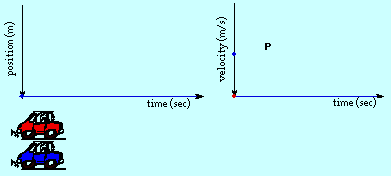# Car Kinematics Question

• Youngstabullz

## Homework Statement

A red car is sitting at a stop light at rest. When the light turns green two things happen simultaneously; (1) the red car accelerates to a speed of 34.3 m/s in 8.92 seconds and then maintains that 34.3 m/s speed and (2) a blue car passes by the red car at a constant speed of 21.5 m/s.

Red Car: Vo=0 Vf=34.3 m/s a=3.84 (34.3/8.92)
Blue Car: Vo=21.5 m/s Vf=21.5 m/s

How much time (in seconds) will have elapsed from when the light turned green until the two cars are side-by-side?## Homework Equations

distance = 1/2(Vo + Vf)t
distance = Vot + 1/2at^2

## The Attempt at a Solution

I thought I would make them equal to each other, 1/2(Vo +Vf)t=Vot +1/2at^2 And find out what t equals to find out the time however It has yet to work after many attempts. Vo Any help is appreciated.

Last edited by a moderator:

Welcome to PF!

A red car is sitting at a stop light at rest. When the light turns green two things happen simultaneously; (1) the red car accelerates to a speed of 34.3 m/s in 8.92 seconds and then maintains that 34.3 m/s speed and (2) a blue car passes by the red car at a constant speed of 21.5 m/s.

Red Car: Vo=0 Vf=34.3 m/s a=3.84 (34.3/8.92)
Blue Car: Vo=21.5 m/s Vf=21.5 m/s

How much time (in seconds) will have elapsed from when the light turned green until the two cars are side-by-side?

I thought I would make them equal to each other, 1/2(Vo +Vf)t=Vot +1/2at^2 And find out what t equals to find out the time however It has yet to work after many attempts.

Hi Youngstabullz! Welcome to PF!Show us your calculations, and then we can see where you've gone wrong.(you have allowed for the period of constant speed of 34.3, haven't you?)

x(blue)=21.5*8.92+21.5*t

x(red)=.5*34.3*8.92+34.3*t

x(blue)=21.5*8.92+21.5*t

x(red)=.5*34.3*8.92+34.3*t

Hi rado5!You must stop giving full answers to other people's questions (you've done it before).

You haven't even given Youngstabullz the chance to reply.On this forum, we try just to give helpful hints, so that the OP can work the problem out themselves.

Full answers should only be given when all hints have failed.

Please restrain yourself.(And your answer was wrong … again!)

Thankfully, I got the answer before you guys had responded to my question. :D

Thankfully, I got the answer before you guys had responded to my question. :D

That's the idea!